# Diagonals

Go back to  'Solid Shapes'

 1 Introduction to Diagonals 2 What is a Diagonal (with illustration)? 3 Think out of the Box! 4 Diagonals of a Square 5 Diagonals of a Triangle 6 Number of Diagonals Formula (with illustration) 7 Length of a Diagonal 8 Important Notes on Diagonals 9 Solved Examples on Diagonals 10 Practice Questions on Diagonals 11 Maths Olympiad Sample Papers 12 Frequently Asked Questions (FAQs)

We at Cuemath believe that Math is a life skill. Our Math Experts focus on the “Why” behind the “What.” Students can explore from a huge range of interactive worksheets, visuals, simulations, practice tests, and more to understand a concept in depth.

Book a FREE trial class today! and experience Cuemath’s LIVE Online Class with your child.

## Introduction to Diagonals

We know that a polygon is a closed shape formed by joining the adjacent vertices.

For example, a square has $$4$$ sides, a pentagon has $$5$$ sides, and a hexagon has $$6$$ sides, and so on.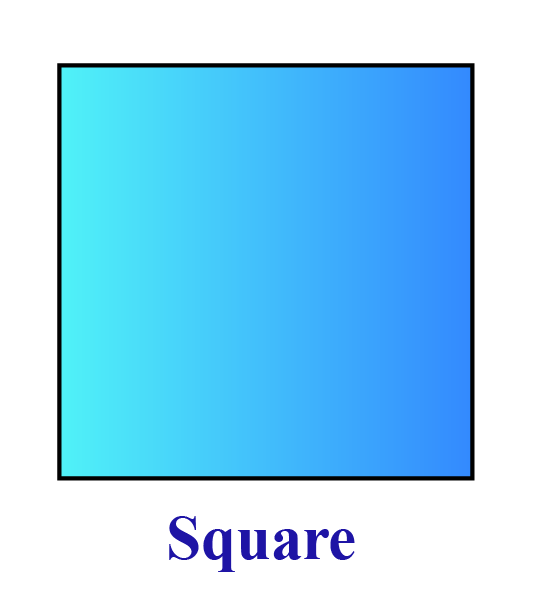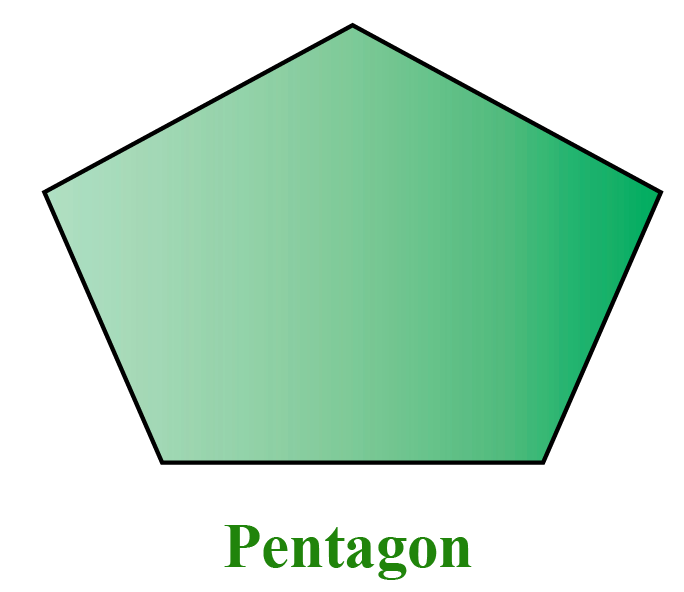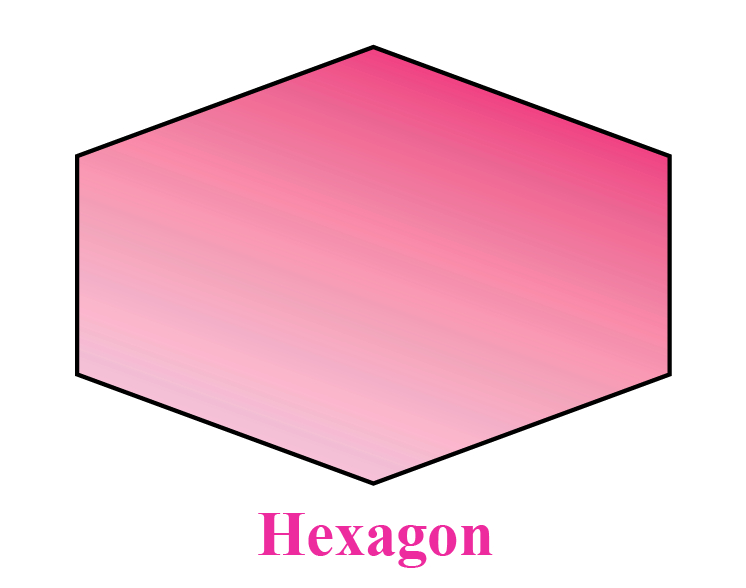Here, we can see that every two adjacent vertices are joined by a line segment.

These line segments are known as the "sides" of the polygon.

But, can we join the non-adjacent vertices by line segments?

If yes, what are such line segments called?

Yes, we can join non-adjacent vertices by line segments.

Such line segments are called diagonals.

Let us study more about diagonals.

## What is a Diagonal (with illustration)?

"A diagonal of a polygon is a line segment that is obtained by joining any two non-adjacent vertices."

Example 1:

Hence, it has two diagonals.

Let us see some quadrilaterals here.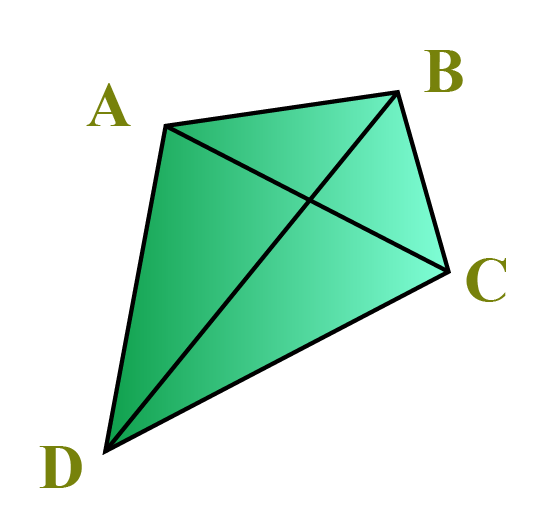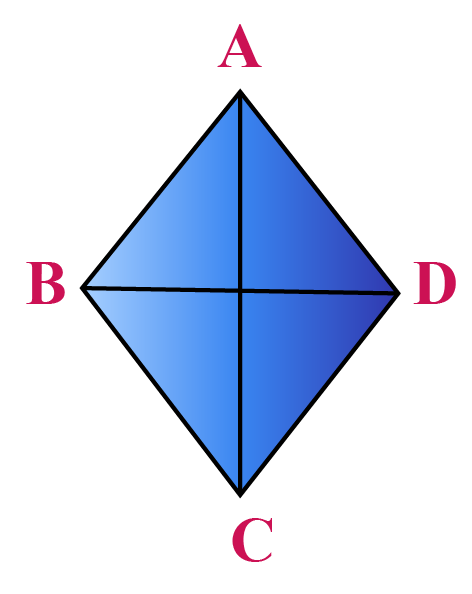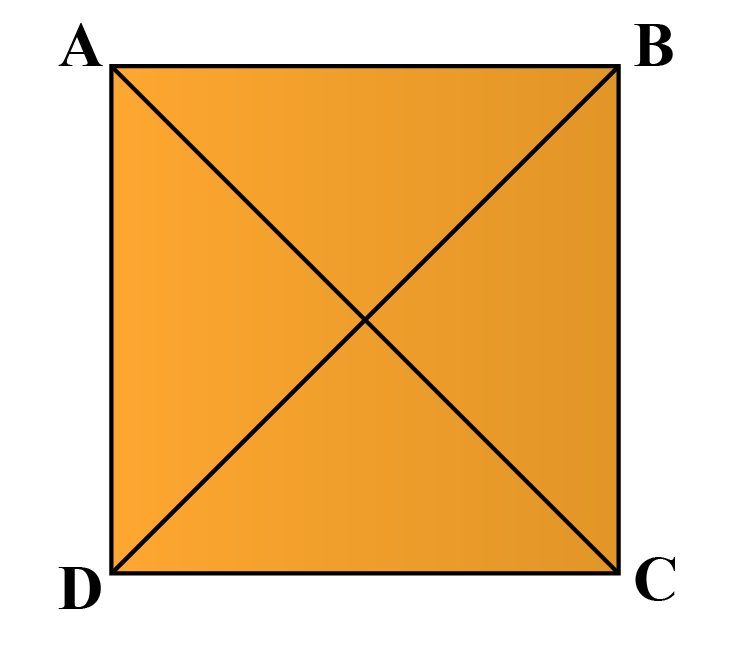In each of these quadrilaterals, $$\overline{AC}$$ and $$\overline{BD}$$ are diagonals.

Example 2:

A hexagon has $$9$$ diagonals.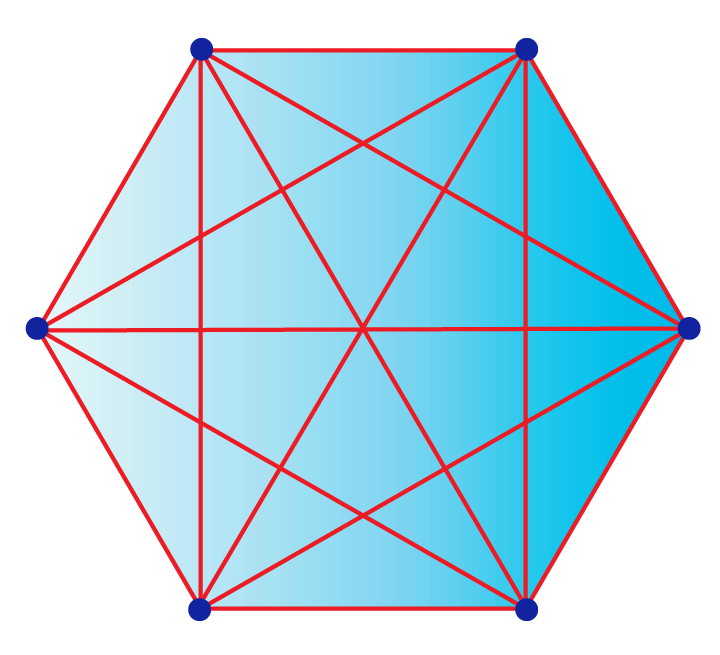Here, all the $$9$$ red-colored lines inside the hexagon are the diagonals.

### Shape of a Diagonal

The shape of a diagonal is a "line segment" and it follows from the definition of a diagonal.

We can see the diagonals of different types of polygons using the following illustration.Think Tank
1. How many diagonals does a circle have?
Tip: Think about the number of vertices of a circle.

## Diagonals of a Square

In a square, there are two pairs of non-adjacent vertices.

By joining the vertices of each such pair, we get two diagonals.In this square $$ABCD$$, the pairs of non-adjacent vertices are $$A$$ and $$C$$, and $$B$$ and $$D$$.

Hence, $$\overline{AC}$$ and $$\overline{BD}$$ are diagonals.

 The number of diagonals of a square = 2

## Diagonals of a Triangle

Let's look at the following triangle $$ABC$$.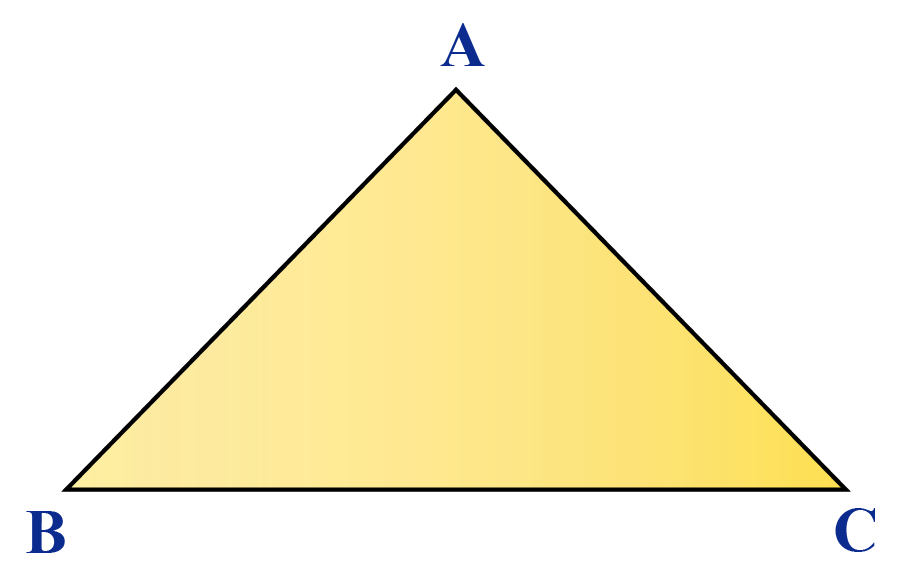Do you see any two vertices of this triangle that are NOT adjacent?

You are right!

No vertices in a triangle are non-adjacent.

It means that there are no line segments which can form diagonals.

 The number of diagonals of a triangle = 0

## Number of Diagonals: Formula (with illustration)

Can you think of the number of diagonals of a heptagon, octagon, nonagon, etc?

We can manually see the number of diagonals of different polygons using the following illustration.

As you can see, it is difficult to draw the diagonals in a polygon with a large number of sides.

As hard as it is to draw them, counting them is equally hard. Don't you agree?

To make this simple, we will use a formula to find the number of diagonals.

The number of diagonals of a polygon with $$\mathbf{n}$$ sides is:

 $$\mathbf{\dfrac{n(n-3)}{2}}$$

We can use this formula to find the number of diagonals of any polygon without actually drawing them.

Example:

Find the number of diagonals of a decagon.

Solution:

The number of sides of a decagon is, $$n=10$$

The number of diagonals of a decagon is calculated using:

\begin{aligned} \dfrac{n(n-3)}{2} &= \dfrac{10(10-3)}{2}\\[0.2cm]&=\dfrac{10(7)}{2}\\[0.2cm]&= \dfrac{70}{2}\\[0.2cm]&=35 \end{aligned}

 The number of diagonals of a decagon= 35

The following table shows the number of diagonals of some polygons which is calculated using this formula.

Shape Number of sides, $$\mathbf{n}$$ Number of Diagonals Formula
Triangle $$3$$

$$\dfrac{3(3-3)}{2}=\color{darkblue}{\boxed{\mathbf{0}}}$$

Quadrilateral $$4$$

$$\dfrac{4(4-3)}{2}=\color{darkblue}{\boxed{\mathbf{2}}}$$

Pentagon $$5$$

$$\dfrac{5(5-3)}{2}=\color{darkblue}{\boxed{\mathbf{5}}}$$

Hexagon $$6$$

$$\dfrac{6(6-3)}{2}=\color{darkblue}{\boxed{\mathbf{9}}}$$

Heptagon $$7$$

$$\dfrac{7(7-3)}{2}=\color{darkblue}{\boxed{\mathbf{14}}}$$

Octagon $$8$$

$$\dfrac{8(8-3)}{2}=\color{darkblue}{\boxed{\mathbf{20}}}$$

Nonagon $$9$$

$$\dfrac{9(9-3)}{2}=\color{darkblue}{\boxed{\mathbf{27}}}$$

Decagon $$10$$

$$\dfrac{10(10-3)}{2}=\color{darkblue}{\boxed{\mathbf{35}}}$$

Hendecagon  $$11$$

$$\dfrac{11(11-3)}{2}=\color{darkblue}{\boxed{\mathbf{44}}}$$

Dodecagon  $$12$$

$$\dfrac{12(12-3)}{2}=\color{darkblue}{\boxed{\mathbf{54}}}$$

## Length of a Diagonal

We have learnt how to find the number of diagonals.

Do you know how to find the length of a diagonal?

Though there are no formulas available to find the length of diagonals for all types of polygons, we have formulas for some specific shapes.

### Length of a Diagonal of a Square

In a square, the length of both the diagonals are the same.

The length of a diagonal ($$d$$) of a square of side length $$x$$ is calculated by the Pythagoras theorem.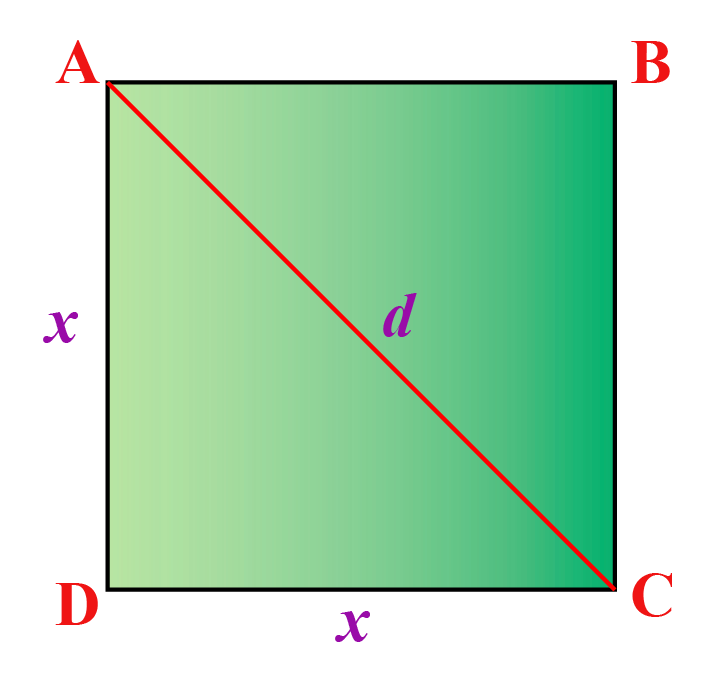Using Pythagoras theorem,

\begin{aligned} d &= \sqrt{x^2+x^2}\\[0.2cm]&= \sqrt{2x^2}\\[0.2cm]&= \sqrt{2} \cdot \sqrt{x^2}\\[0.2cm] &=\sqrt{2}\,\,x \end{aligned}

 Length of a diagonal of a square = $$\mathbf{\sqrt{2}\,\,x }$$

### Length of a Diagonal of a Rectangle

Similar to a square, the length of both the diagonals in a rectangle are the same.

The length of a diagonal ($$d$$) of a rectangle whose length is $$l$$ and whose breadth is $$b$$ is calculated by the Pythagoras theorem.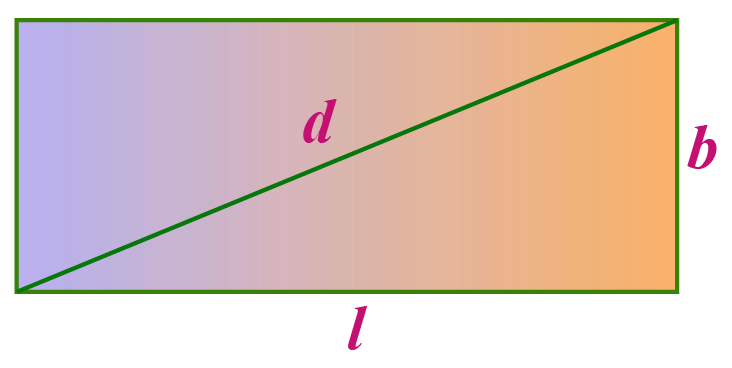Using Pythagoras theorem,

\begin{aligned} d &= \sqrt{l^2+b^2} \end{aligned}

 Length of a diagonal of a rectangle = $$\mathbf{\sqrt{l^2+b^2}}$$

### Length of a Diagonal of a Cube

Consider a cube of length $$x$$

A cube has $$6$$ faces.

Each face of a cube is a square.

Thus each face has two diagonals.

Hence, the length of each such diagonal is the same as the length of a diagonal of a square.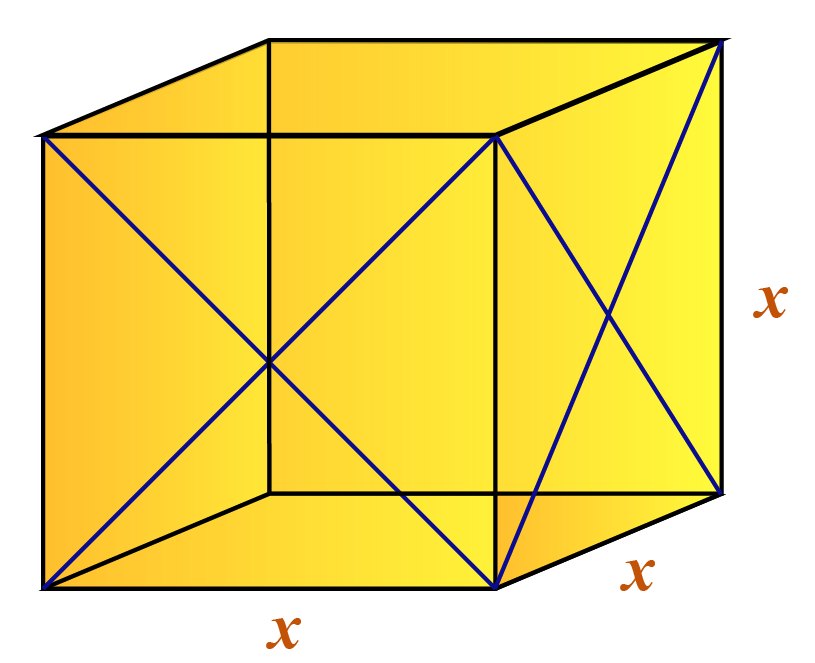Length of each face diagonal of cube = $$\mathbf{\sqrt{2}\,\,x }$$

Apart from the diagonals on the faces, there are $$4$$ other diagonals (main diagonals or body diagonals) that pass through the center of the square.

Let us assume that the length of each such diagonal is $$d$$.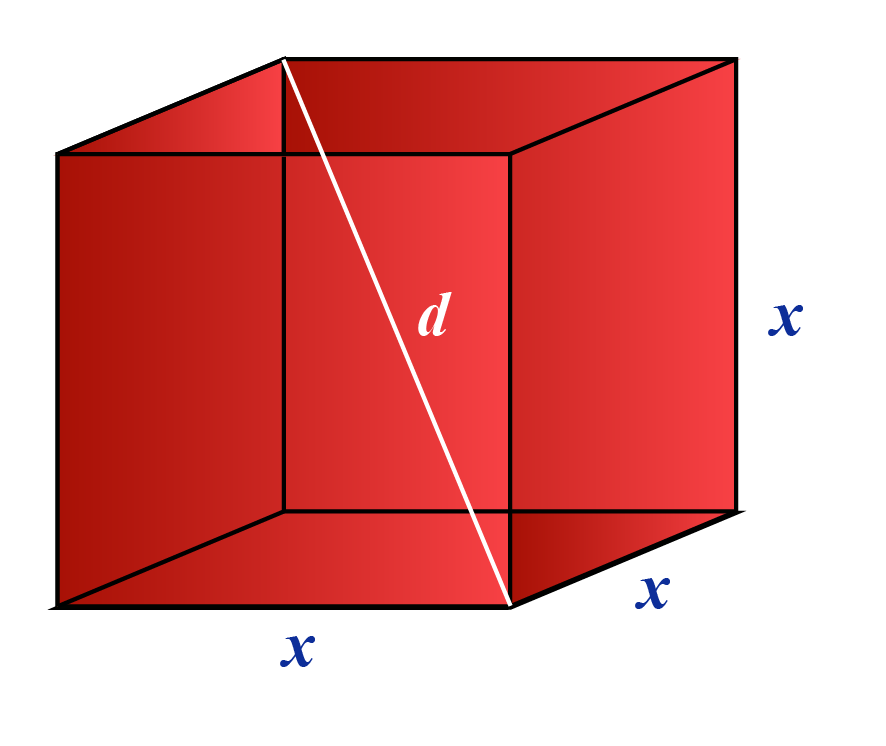The formula for the length of the diagonal of a cube is derived in the same way as we derive the length of the diagonal of a square.

 Length of a diagonal of a cube = $$\mathbf{\sqrt{3}\,\,x }$$

### Length of a Diagonal of a Cuboid (Rectangular Prism)

Consider a cuboid of length $$l$$, width $$w$$ and height $$h$$.

Let us assume that its main diagonal (or body diagonal) that passes through the center of the cuboid is $$d$$.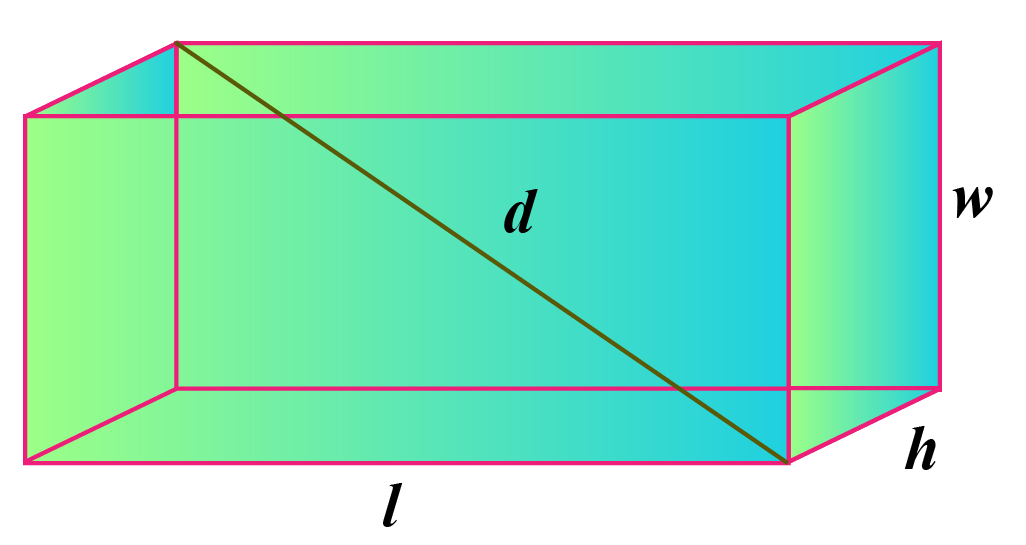The number of diagonals of a polygon with $$\mathbf{n}$$ sides is

$$\mathbf{\dfrac{n(n-3)}{2}}$$

 Length of a diagonal of a cuboid = $$\mathbf{\sqrt{l^2+w^2+h^2} }$$

Help your child score higher with Cuemath’s proprietary FREE Diagnostic Test. Get access to detailed reports, customised learning plans and a FREE counselling session. Attempt the test now.

## Solved Examples

 Example 1

If a polygon has $$90$$ diagonals, how many sides does it have?

Solution:

Let us assume that the number of sides of the given polygon is $$n$$.

The number of diagonals = $$90$$

\begin{aligned} \dfrac{n(n-3)}{2}&= 90 \\[0.2cm] n(n-3) &=180\\[0.2cm] n^2-3n-180&=0\\[0.2cm] (n-15)(n+12)&=0\\[0.2cm] n=15; & n=-12 \end{aligned}

Since $$n$$ cannot be negative, the value of $$n$$ is $$15$$.

 $$\therefore$$ Sides of the given polygon = $$15$$
 Example 2

A cube has a wall area of $$121 \text{ cm}^2$$.

What is the length of the main diagonal of the cube?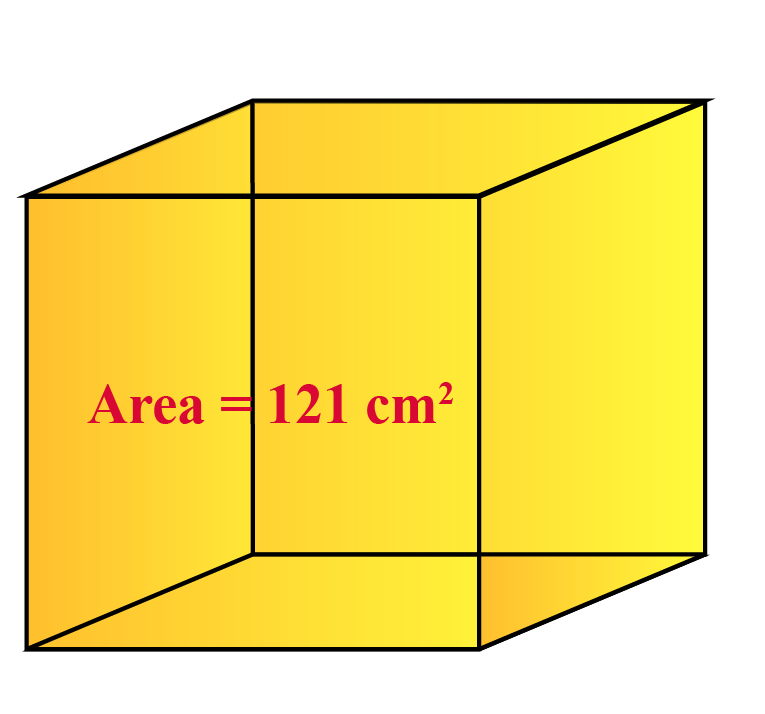Solution:

Let us assume that $$x$$ cm is the side of the given cube.

Its wall is a square.

Thus, the area of the wall = $$x^2 \text{ cm}^2$$

Since the area of the wall is $$121 \text{ cm}^2$$,

\begin{aligned} x^2 &=121\\[0.2cm] x&=11 \end{aligned}

Let us assume that the length of the diagonal of the cube is $$d$$ cm.

This implies,

$d = \sqrt{3} \,\, x = \sqrt{3} \,\, (11) = 11 \sqrt{3} \text { cm}$

 $$\therefore$$ Length of the main diagonal = $$\ 11 \sqrt{3} \text { cm}$$
 Example 3

The size of a television screen is nothing but the length of its diagonal.

If the dimensions of a television are $$16$$ inches and $$40$$ inches, find the size of the television.

Solution:

We know that a television is in the shape of a rectangle.

Length $$l = 40$$ inches

Breadth $$b =16$$ inches

The size of the television is the length of its diagonal length and we will assume it to be $$d$$.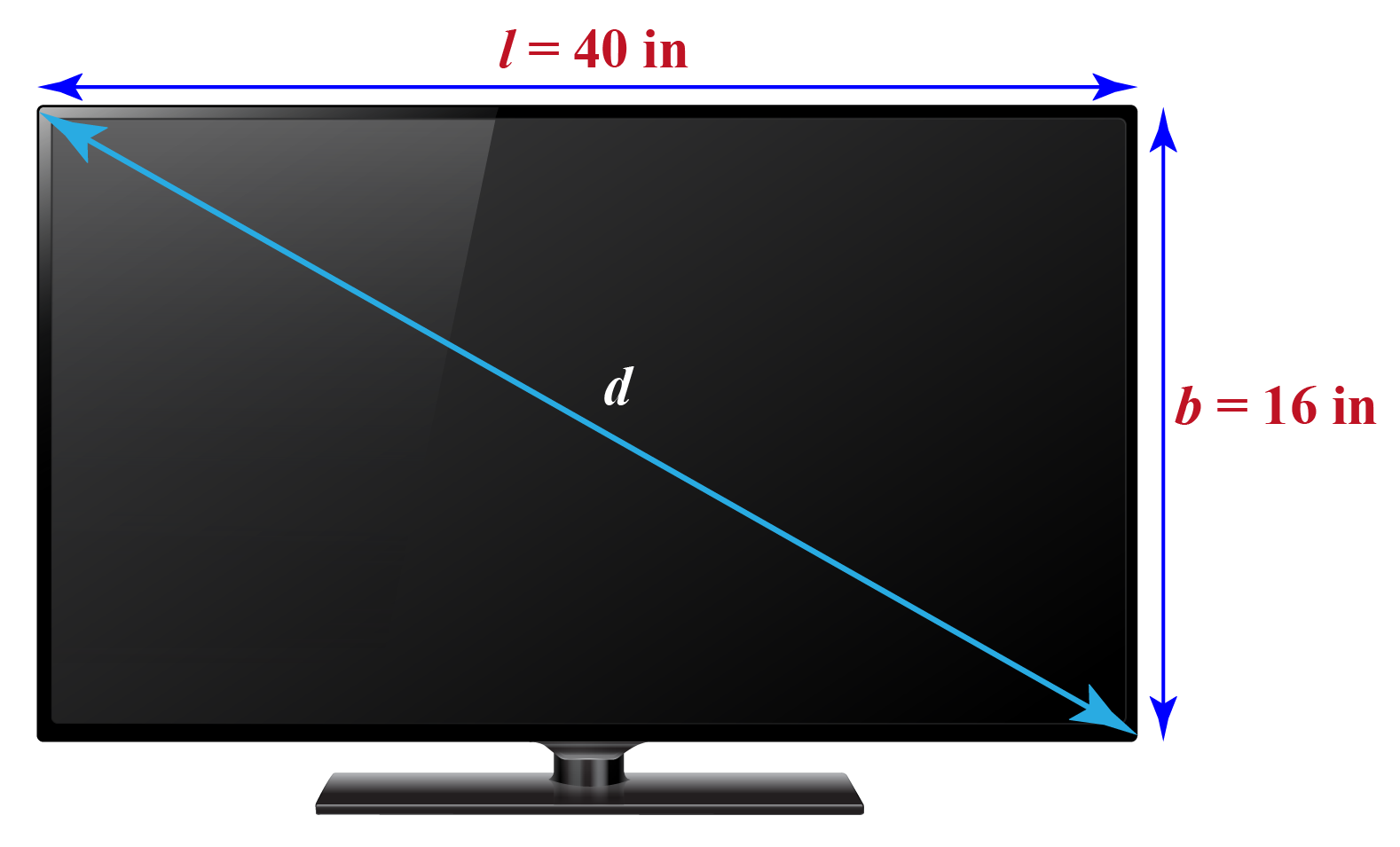We know that,

\begin{aligned} d&= \sqrt{l^2+b^2}\\[0.2cm] &= \sqrt{40^2+16^2}\\[0.2cm] &= \sqrt{1856}\\[0.2cm] & \approx 43.08 \text{ inches} \end{aligned}

 $$\therefore$$ Size of the television = $$43.08 \text { inches}$$

CLUEless in Math? Check out how CUEMATH Teachers will explain Diagonals to your kid using interactive simulations & worksheets so they never have to memorise anything in Math again!

Explore Cuemath Live, Interactive & Personalised Online Classes to make your kid a Math Expert. Book a FREE trial class today!

## Practice Questions

Here are a few activities for you to practice.

IMO (International Maths Olympiad) is a competitive exam in Mathematics conducted annually for school students. It encourages children to develop their math solving skills from a competition perspective.

## 1. What is the angle of a diagonal in a square?

Each diagonal in a square divides the angle at each vertex into two equal parts.

Hence, the angle between any side and any diagonal is 45 degrees.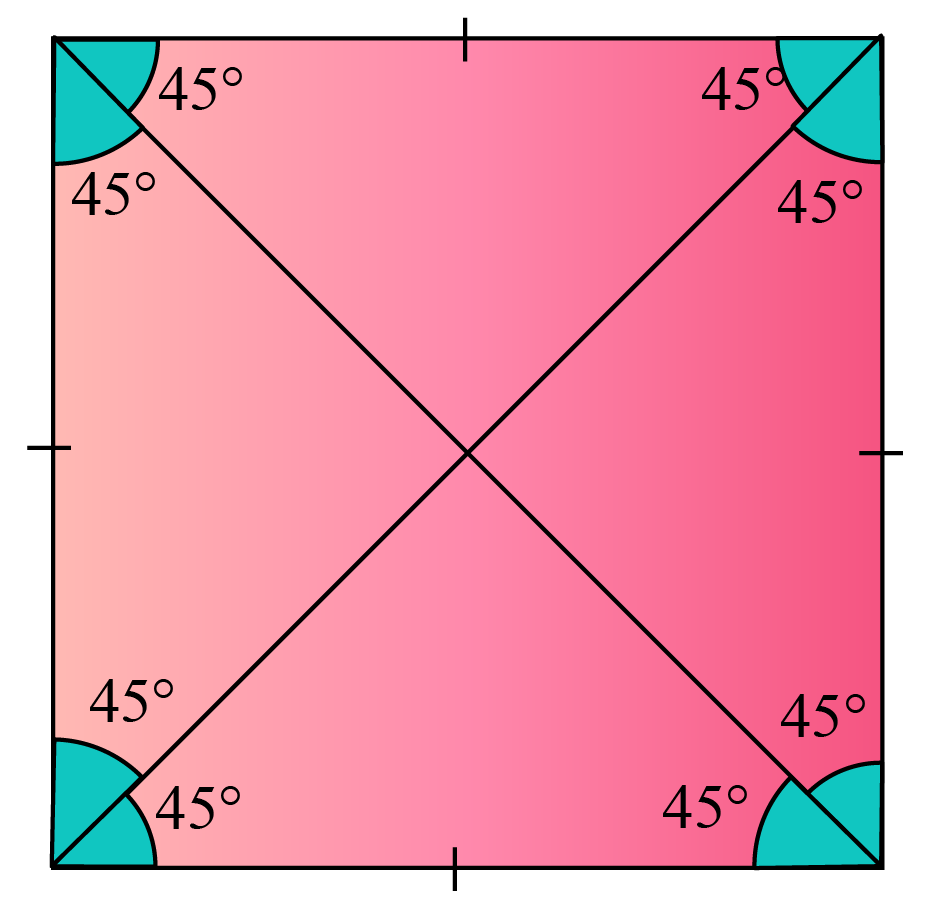## 2. What do you mean by diagonals?

A diagonal of a polygon is a line segment that is obtained by joining any two non-adjacent vertices.

## 3. What does a diagonal look like?

The shape of a diagonal is a line segment.

## 4. How many sides does a diagonal have?

A diagonal itself is a line segment.

Thus, a diagonal cannot have any sides.

More Important Topics
Numbers
Algebra
Geometry
Measurement
Money
Data
Trigonometry
Calculus